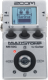# Wild_JCM2k

Discussion in 'Zoom MS-50G' started by Zakhar Parov, Dec 6, 2018.

1. Wild_JCM2kDevice: Zoom Ms-50g
Firmware: 3.00

Name on device: Wild_JCM2k
Optimized for: Guitar Amp

Effects chain:The high gain sound of a JCM2000 Marshall stack amp.

Effect: "Z Wild" (Overdrive / Distortion), active - "yes"
"Gain" = 60
"Tone" = 70
"Level" = 5

Effect: "MS DRIVE" (Amp simulator), active - "yes"
"Gain" = 26
"Tube" = 12
"Level" = 30
"Trebl" = 44
"Middl" = 24
"Bass" = 76
"Prese" = 56
"CAB" = HW STACK 4x12
"OUT" = STACK POWER AMP

Effect: "ParaEQ" (Dynamics / Filter), active - "yes"
"Freq1" = 80Hz
"Q1" = 1
"Gain1" = 4
"Freq2" = 6.3kHz
"Q2" = 1
"Gain2" = 2
"Level" = 100

Effect: "ParaEQ" (Dynamics / Filter), active - "yes"
"Freq1" = 400Hz
"Q1" = 2
"Gain1" = -3
"Freq2" = 800Hz
"Q2" = 2
"Gain2" = -5
"Level" = 100

Effect: "ZNR" (Dynamics / Filter), active - "yes"
"THRSH" = 2
"DETCT" = GtrIn
"Level" = 100

Effect: "HD Reverb" (Delay / Reverb), active - "yes"
"Decay" = 20
"Tone" = 6
"Mix" = 4
"PreD" = 42
"HPF" = 4
"Level" = 100
"Tail" = Off

Note: This is a patch file, you will need to download and install the ToneLib software to use the patch.

File size:
467 bytes
Views:
228